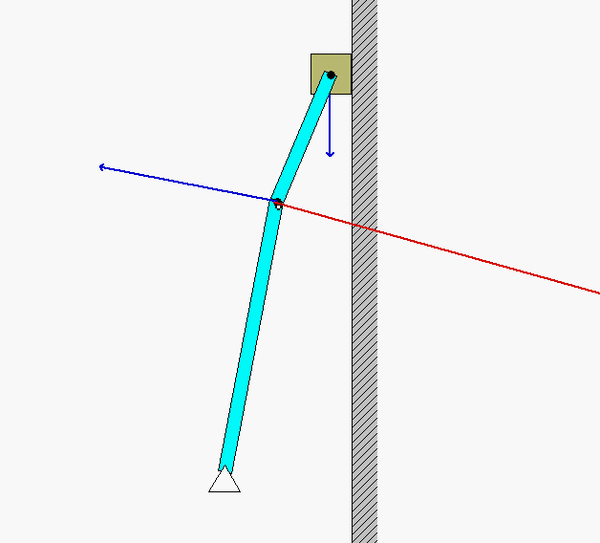# Homework H.2.DAnimation of motionNote that as link BD passes through the horizontal orientation, the velocity of B (as well as the angular velocity of AB) instantaneously goes to zero, yet the acceleration of B is not zero. In a couple days when we cover instant centers of zero velocity we will be able to predict when point B has zero velocity.

Solution plan

1. Note that since D is traveling on a straight line with constant speed, the acceleration of D is zero. Therefore, we can write: a_B = a_D + alpha_BD x r_B/D - omega_BD^2*r_B/D = alpha_BD x r_B/D - omega_BD^2*r_B/D.
2. Note that since A is a pin joint, the acceleration of A is zero. Therefore, we can write: a_B = a_A + alpha_AB x r_B/A - omega_AB^2*r_B/A = alpha_AB x r_B/A - omega_AB^2*r_B/A.
3. Equate the expressions of a_B  from 1. and 2. above. This gives you two SCALAR equations in order to solve for two unknowns, alpha_AB and alpha_BD.

Note that you need to solve the velocity problem first. Follow the above procedure to do so. Here. the velocity of D is known as v_D = -v_D*j_hat.